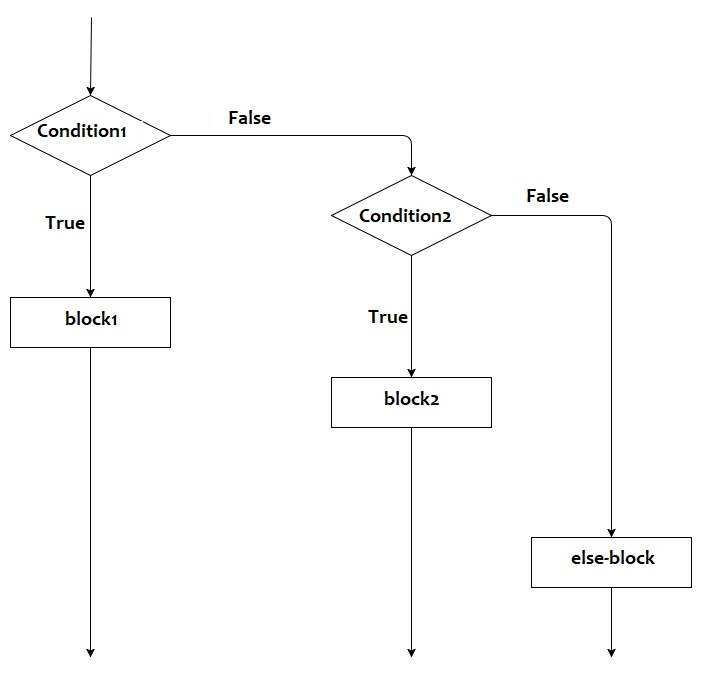Submitted by IncludeHelp, on December 10, 2021

The if-else-if ladder statement is used to execute one block from the given multiple blocks if any condition is met. The if-else-if ladder statement has more than one condition and executes from top to down. If condition1 is true then block1 (set of the statements associated with the condition1 statement) will be executed, if condition2 is false then condition2 will be checked if it is true then block2 (set of the statements associated with the condition2 statement) will be executed, if condition2 is false then next condition will be checked and so on. If all conditions are false then else-block will be executed.

Syntax:

```if(condition1){
// block1
}else if(condition2){
// block2
}...{
.
.
.
}else{
//else-block
}

or

if condition1 {
// block1
} else if condition2{
// block2
}...{
.
.
.
}else{
//else-block
}
```

Flow chart:Example 1: Input percentage and check the division (>=60% - First division, >=50 - second division, >=40 - Third division, else fail).

In this program, we will use the if-else-if ladder condition to check the division.

```// Golang program to demonstrate the
// example of the if-else-if ladder statement

package main

import "fmt"

func main() {
var perc float32

fmt.Print("Input the percentage: ")
fmt.Scanf("%f", &perc)

if perc >= 60 {
fmt.Println("First division.")
} else if perc >= 50 {
fmt.Println("Second division.")
} else if perc >= 40 {
fmt.Println("Third division.")
} else {
fmt.Println("Fail.")
}
}
```

Output:

```RUN 1:
Input the percentage: 84.9
First division.

RUN 2:
Input the percentage: 54
Second division.

RUN 3:
Input the percentage: 42
Third division.

RUN 4:
Input the percentage: 34
Fail.
```

Example 2: Input an integer value to check whether the input number is positive, negative, or zero.

In this program, we will use the if-else-if ladder statement to check whether the input number is positive, negative, or zero.

```// Golang program to demonstrate the
// example of the if-else-if ladder statement

package main

import "fmt"

func main() {
var num int

fmt.Print("Input an integer number: ")
fmt.Scanf("%d", &num)

if num > 0 {
fmt.Println("Number is positive.")
} else if num < 0 {
fmt.Println("Number is negative.")
} else {
fmt.Println("Number is zero.")
}
}
```

Output:

```RUN 1:
Input an integer number: 108
Number is positive.

RUN 2:
Input an integer number: -54
Number is negative.

RUN 3:
Input an integer number: 0
Number is zero.
```

Preparation

What's New

Top Interview Coding Problems/Challenges!